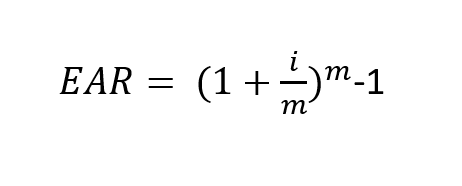# Equivalent Annual Rate

This adjusts a nominal interest rate by how many times over the course of a year compounding occurs, in order to give us a more accurate interest rate expressed in annual terms. Given by the equation:where:

EAR = equivalent annual rate

i = nominal interest rate

m = number of compounding periods per year